Publicité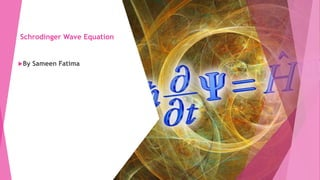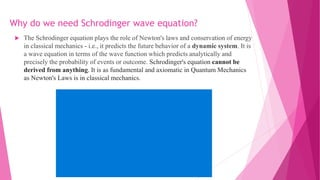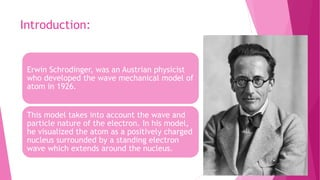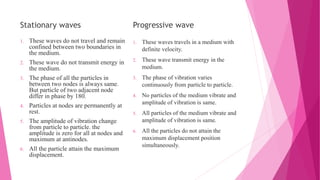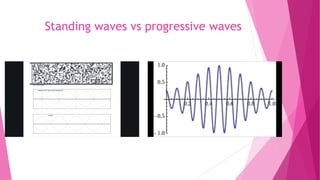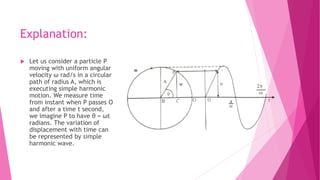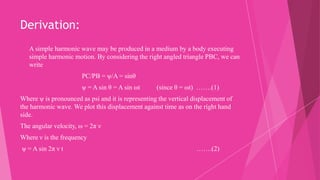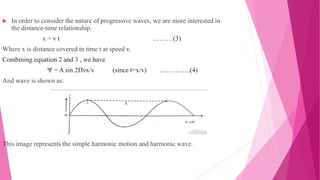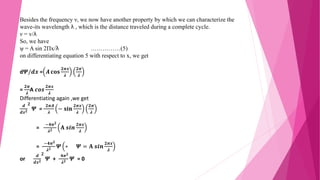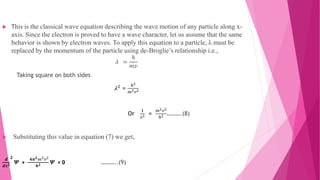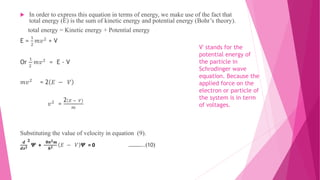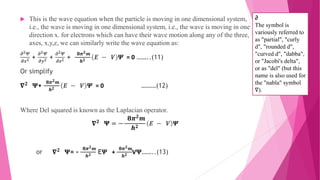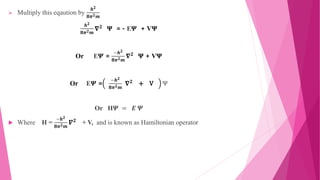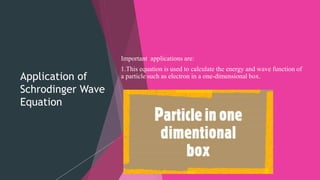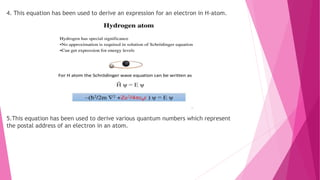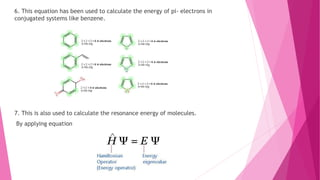1 sur 20
Publicité

### Schrodinger wave equation.pptx

1. Schrodinger Wave Equation By Sameen Fatima
2. Why do we need Schrodinger wave equation?  The Schrodinger equation plays the role of Newton's laws and conservation of energy in classical mechanics - i.e., it predicts the future behavior of a dynamic system. It is a wave equation in terms of the wave function which predicts analytically and precisely the probability of events or outcome. Schrodinger's equation cannot be derived from anything. It is as fundamental and axiomatic in Quantum Mechanics as Newton's Laws is in classical mechanics.
3. In physical chemistry, a dynamical system is described as a "particle or ensemble of particles whose state varies over time and thus obeys differential equations involving time derivatives". In order to make a prediction about the system's future behavior, an analytical solution of such equations or their integration over time through computer simulation is realized.
4. Introduction: Erwin Schrodinger, was an Austrian physicist who developed the wave mechanical model of atom in 1926. This model takes into account the wave and particle nature of the electron. In his model, he visualized the atom as a positively charged nucleus surrounded by a standing electron wave which extends around the nucleus.
5. Stationary waves 1. These waves do not travel and remain confined between two boundaries in the medium. 2. These wave do not transmit energy in the medium. 3. The phase of all the particles in between two nodes is always same. But particle of two adjacent node differ in phase by 180. 4. Particles at nodes are permanently at rest. 5. The amplitude of vibration change from particle to particle. the amplitude is zero for all at nodes and maximum at antinodes. 6. All the particle attain the maximum displacement. Progressive wave 1. These waves travels in a medium with definite velocity. 2. These wave transmit energy in the medium. 3. The phase of vibration varies continuously from particle to particle. 4. No particles of the medium vibrate and amplitude of vibration is same. 5. All particles of the medium vibrate and amplitude of vibration is same. 6. All the particles do not attain the maximum displacement position simultaneously.
6. Standing waves vs progressive waves
7. Explanation:  Let us consider a particle P moving with uniform angular velocity ω rad/s in a circular path of radius A, which is executing simple harmonic motion. We measure time from instant when P passes O and after a time t second, we imagine P to have θ = ωt radians. The variation of displacement with time can be represented by simple harmonic wave.
8. Derivation:  A simple harmonic wave may be produced in a medium by a body executing simple harmonic motion. By considering the right angled triangle PBC, we can write PC/PB = ψ/A = sinθ ψ = A sin θ = A sin ωt (since θ = ωt) …….(1) Where ψ is pronounced as psi and it is representing the vertical displacement of the harmonic wave. We plot this displacement against time as on the right hand side. The angular velocity, ω = 2π ν Where ν is the frequency ψ = A sin 2π ν t …….(2)
9.  In order to consider the nature of progressive waves, we are more interested in the distance-time relationship. x = v t ………(3) Where x is distance covered in time t at speed v. Combining equation 2 and 3 , we have Ψ = A sin 2Πνx/v (since t=x/v) …………..(4) And wave is shown as: This image represents the simple harmonic motion and harmonic wave.
10. Besides the frequency ν, we now have another property by which we can characterize the wave-its wavelength λ , which is the distance traveled during a complete cycle. ν = v/λ So, we have ψ = A sin 2Πx/λ ……………(5) on differentiating equation 5 with respect to x, we get d𝜳/𝒅𝒙 = 𝑨 𝐜𝐨𝐬 𝟐𝝅𝒙 𝝀 𝟐𝝅 𝝀 = 𝟐𝝅 𝝀 A 𝒄𝒐𝒔 𝟐𝝅𝒙 𝝀 Differentiating again ,we get 𝒅 𝒅𝒙𝟐 𝟐 𝜳 = 𝟐𝝅𝑨 𝝀 − 𝐬𝐢𝐧 𝟐𝝅𝒙 𝝀 𝟐𝝅 𝝀 = −𝟒𝝅𝟐 𝝀𝟐 𝐀 𝒔𝒊𝒏 𝟐𝝅𝒙 𝝀 = −𝟒𝝅𝟐 𝝀𝟐 𝜳 ∗ 𝜳 = 𝐀 𝒔𝒊𝒏 𝟐𝝅𝒙 𝝀 or 𝒅 𝒅𝒙𝟐 𝟐 𝜳 + 𝟒𝝅𝟐 𝝀𝟐 𝜳 = 0
11.  This is the classical wave equation describing the wave motion of any particle along x- axis. Since the electron is proved to have a wave character, let us assume that the same behavior is shown by electron waves. To apply this equation to a particle, λ must be replaced by the momentum of the particle using de-Broglie’s relationship i.e., 𝜆 = ℎ 𝑚𝑣 Taking square on both sides 𝝀𝟐 = 𝒉𝟐 𝒎𝟐𝒗𝟐 Or 𝟏 𝝀𝟐 = 𝒎𝟐𝒗𝟐 𝒉𝟐 ……….(8)  Substituting this value in equation (7) we get, 𝒅 𝒅𝒙𝟐 𝟐 𝜳 + 𝟒𝝅𝟐𝒎𝟐𝒗𝟐 𝒉𝟐 𝜳 = 0 ………..(9)
12.  In order to express this equation in terms of energy, we make use of the fact that total energy (E) is the sum of kinetic energy and potential energy (Bohr’s theory). total energy = Kinetic energy + Potential energy E = 1 2 𝑚𝑣2 + V Or 1 2 𝑚𝑣2 = E - V 𝑚𝑣2 = 2 𝐸 − 𝑉 𝑣2 = 2 𝐸 − 𝑉 𝑚 Substituting the value of velocity in equation (9). 𝒅 𝒅𝒙𝟐 𝟐 𝜳 + 𝟖𝝅𝟐𝒎 𝒉𝟐 𝐸 − 𝑉 𝜳 = 0 ………..(10) V' stands for the potential energy of the particle in Schrodinger wave equation. Because the applied force on the electron or particle of the system is in term of voltages.
13.  This is the wave equation when the particle is moving in one dimensional system, i.e., the wave is moving in one dimensional system, i.e., the wave is moving in one direction x. for electrons which can have their wave motion along any of the three, axes, x,y,z, we can similarly write the wave equation as: 𝝏𝟐𝜳 𝝏𝒙𝟐 + 𝝏𝟐𝜳 𝝏𝒚𝟐 + 𝝏𝟐𝜳 𝝏𝒛𝟐 + 𝟖𝝅𝟐𝒎 𝒉𝟐 𝑬 − 𝑽 𝜳 = 0 ……..(11) Or simplify 𝛁𝟐 𝚿+ 𝟖𝝅𝟐𝒎 𝒉𝟐 𝑬 − 𝑽 𝜳 = 0 ………(12) Where Del squared is known as the Laplacian operator. 𝛁𝟐 𝚿 = − 𝟖𝝅𝟐 𝒎 𝒉𝟐 𝑬 − 𝑽 𝜳 or 𝛁𝟐 𝚿= - 𝟖𝝅𝟐𝒎 𝒉𝟐 E𝜳 + 𝟖𝝅𝟐𝒎 𝒉𝟐 V𝚿……..(13) ∂ The symbol is variously referred to as "partial", "curly d", "rounded d", "curved d", "dabba", or "Jacobi's delta", or as "del" (but this name is also used for the "nabla" symbol ∇).
14.  Multiply this eqaution by 𝒉𝟐 𝟖𝝅𝟐𝒎 𝒉𝟐 𝟖𝝅𝟐𝒎 𝛁𝟐 𝚿 = - E𝜳 + V𝚿 Or E𝜳 = −𝒉𝟐 𝟖𝝅𝟐𝒎 𝛁𝟐 𝚿 + V𝚿 Or E𝜳 = −𝒉𝟐 𝟖𝝅𝟐𝒎 𝛁𝟐 + V Ψ Or H𝜳 = 𝑬 𝜳  Where H = −𝒉𝟐 𝟖𝝅𝟐𝒎 𝜵𝟐 + V, and is known as Hamiltonian operator
15. Application of Schrodinger Wave Equation Important applications are: 1.This equation is used to calculate the energy and wave function of a particle such as electron in a one-dimensional box.
16. 2.This equation is used to calculate the energy and wave function of a particle in a three-dimensional box.
17. 3. This equation introduces the concept of degeneracy in atomic orbitals.
18. 4. This equation has been used to derive an expression for an electron in H-atom. 5.This equation has been used to derive various quantum numbers which represent the postal address of an electron in an atom.
19. 6. This equation has been used to calculate the energy of pi- electrons in conjugated systems like benzene. 7. This is also used to calculate the resonance energy of molecules. By applying equation
Publicité